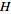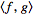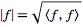# Complete sets and complete spaces

• I
• cromata
The norm may be induced by an inner product as in Hilbert spaces, but need not be.In summary, a complete space is one in which every Cauchy sequence has a limit within the space, and from this definition, we can define a Hilbert space. A Hilbert space is a complete vector space with an inner product that also turns it into a complete metric space. A complete set is an orthonormal set of vectors in an inner product space, and it is said to be complete if every element in the space can be expressed as a finite or infinite sum of the inner product of the vectors in the set. In infinite dimensional inner vector spaces, an infinite set of orthonormal vectors is not necessarily a completef

#### cromata

-Definition of complete space: if every Cauchy sequence of points in M has a limit that is also in M or, alternatively, if every Cauchy sequence in converges in M. (and from this definition we can define Hilbert Space)
-Definition of Hilbert space:
A Hilbert space is a vector spacewith an inner productsuch that the norm defined byturnsinto a complete metric space
-Definition of complete set: If V is inner product space and {ek}k∈ℕ is orthonormal set of vectors then we say that {ek}k∈ℕ is complete if for every u∈V u=∑<ek,u>ek

I have several questions:
-In infinite dimension inner vector spaces, infinite set of orthonormal vectors is not necessarily complete vector set? (is this true, and if it is, why?)

-Are complete sets and complete vector spaces connected in some way? Is every infinite set of orthonormal vectors in complete space (or more precisely in Hilbert space) complete set?

-What is the difference between complete set and basis?

#### Attachments

Definition of complete set: If V is inner product space and {ek}k∈ℕ is orthonormal set of vectors then we say that {ek}k∈ℕ is complete if for every u∈V u=∑<ek,u>ek
This contains a critical ambiguity - that you have not specified whether the sum is finite or infinite.
An orthonormal basis for a Hilbert space H is an orthonormal set of vectors whose span is dense in H.
An orthonormal Hamel basis for a Hilbert space H is an orthonormal set of vectors whose span is equal to H.
-In infinite dimension inner vector spaces, infinite set of orthonormal vectors is not necessarily complete vector set? (is this true, and if it is, why?)
Say ##\{e_k\}_{k\in\mathbb N}## is a basis. Then the infinite, orthonormal subset ##\{e_{2k}\}_{k\in\mathbb N}## of even-numbered elements of the set, is not complete (because if it were the original set would not be linearly independent), and hence not a basis.

-What is the difference between complete set and basis?
As I learned it, completeness of a set of vectors does not entail linear independence. If a complete set is not linearly independent, it is not a basis. But your definition above includes orthonormality, which entails linear independence, so your definition looks the same as that of either an orthonormal Hamel basis (if the sums must be finite) or an orthonormal basis (if they can be infinite).

•cromata
These definitions are not restricted to Hilbert spaces. They apply to any vector space, where there is a defined norm.